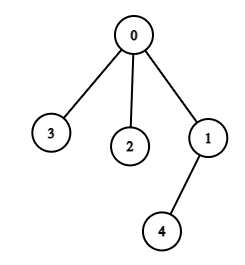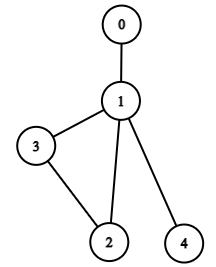0

# Graph Valid Tree

Difficulty: MEDIUMContributed By
Ankit Kharb
Avg. time to solve
30 min
Success Rate
70%

Problem Statement

#### Tree is a special graph. It has the following properties.

``````• It is connected
• It has no cycle.
``````

#### Example :

``````If n = 5 and if there are 4 edges i.e [ [0, 1], [0, 2], [0, 3], [1, 4]] so the graph formed will look like this:-
````````````Here this graph is connected and it has no cycle so it is a tree.
``````
##### Input Format :
``````The first line of input contains an integer T’ denoting the number of test cases to run. Then the test case follows.

The first line of each test contains an integer ‘N’ where N is the number of nodes.

The next line of each test case contains an integer ‘M’ where M is the number of edges.

Then the following m lines contain two space separated integers each representing the list of undirected edges.
``````
##### Output Format :
``````For each test case, print "True" if the graph is a valid tree otherwise print "False".
``````
##### Note :
``````You don’t have to take input or print anything. This already has been taken care of. Just implement the function.
``````
##### Constraints :
``````1 <= T <= 5
1 <= N <= 10^3
1 <= M <= 10^3
``````
##### Sample Input 1 :
``````2
5
4
0 1
0 2
0 3
1 4
5
5
0 1
1 2
2 3
1 3
1 4
``````
##### Sample Output 1 :
``````True
False
``````
##### Explanation of sample input 1 :
``````Test case 1:

If n = 5, and k = 5 and undirected edges are [0, 1], [0, 2], [0, 3], [1, 4]. With these edges the graph will look like this:
````````````Here there is no cycle and the graph is connected hence it is a tree.

Test case 2:

If n = 5 and k = 5 and the edges are [0, 1], [1, 2], [2, 3], [1, 3], [1, 4]. The graph will look like this:
````````````Here there is a cycle between nodes 1,2 and 3. Hence this graph is not a tree.
``````
##### Sample Input 2 :
``````2
5
5
0 1
0 2
1 3
3 2
2 4
4
3
0 1
0 2
1 3
``````
##### Sample Output 2 :
``````False
True
``````Console